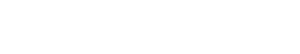# Collocation

Collocation is a term used in text analytics to refer to the grouping of words that often occur together. This can be thought of as a type of statistical association between words, where the co-occurrence of two or more words is greater than would be expected by chance.

Collocation is similiar to, but not the same as, terms such as n-grams and keywords. N-grams are simply a sequence of n items from a given text, where n can be any number. Keywords are words that are associated with a particular topic. In contrast, collocation is more specific, referring to the statistical association between words.

Collocation can be useful for understanding the meaning of a text, as well as for identifying potential keywords and topics. It can also be used for predictive modeling, such as for identifying the likelihood of certain words occurring together.

## Methods of Collocation

There are a variety of methods for finding collocations in a text, including simple counts, pointwise mutual information, and log-likelihood ratios.

Simple counts involve simply counting the number of times that two words occur together. This can be done using a co-occurrence matrix, which is a table that shows the number of times each word occurs with every other word in the text.

Pointwise mutual information (PMI) is another method for calculating the strength of association between two words. PMI is based on the idea that if two words are more likely to occur together than would be expected by chance, then they have a strong association. The PMI value for two words can be calculated as:

PMI = log2 (P(w1, w2) / P(w1) * P(w2))

where P(w1, w2) is the probability of the two words occurring together, and P(w1) and P(w2) are the probabilities of the words occurring separately.

Log-likelihood ratio (LLR) is another method for calculating the strength of association between two words. LLR is based on the idea that if two words are more likely to occur together than would be expected by chance, then they have a strong association. The LLR value for two words can be calculated as:

LLR = 2 * log (P(w1, w2) / P(w1) * P(w2))

where P(w1, w2) is the probability of the two words occurring together, and P(w1) and P(w2) are the probabilities of the words occurring separately.

## Applications of Collocation

There are a variety of ways in which collocation can be used. In general, collocation can be helpful for understanding the meaning of a text, as well as for identifying potential keywords and topics. It can also be used for predictive modeling, such as for identifying the likelihood of certain words occurring together.

Some specific applications of collocation include:

• Identifying synonyms: Collocation can be used to identify words that have the same meaning, or that are related to each other in some way. For example, the words “car” and “automobile” are considered to be synonyms, as they refer to the same thing.
• Disambiguating words: Collocation can also be used to disambiguate words that have multiple meanings. For example, the word “bank” can refer to a financial institution, or it can refer to the edge of a river. By looking at the context in which the word is used, collocation can help to determine which meaning is intended.
• Improved information retrieval: Collocation can be used to improve information retrieval, as it can help to identify the most relevant documents for a given query. For example, if a user searches for the term “car”, documents that contain the collocates “automobile”, “vehicle”, or “transportation” are more likely to be relevant than those that do not contain these terms.Unlock the power of actionable insights with AI-based natural language processing.